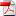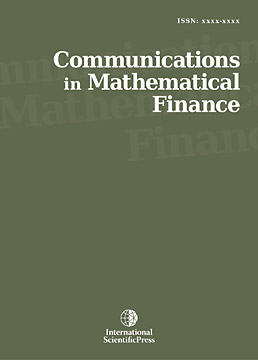# Communications in Mathematical Finance

#### Arithmetic Moving Average and Optimization of Expected Utility of Wealth

•[ Download ]
• Abstract

From the perspective of asset allocation, the moving average trading method is studied by providing the complete optimal investment solution for the expected log-utility of wealth under the arithmetic moving average (AMA) rule. The technical analysis adds value to the practical fixed allocation rules if stock returns are not predictable. We also show that the implement approximation for the optimal strategy can be constructed explicitly and is convergent to the theoretical optimal investment solution for the AMA. We illustrate numerically that the geometric moving average (GMA) rule can either overestimate or underestimate the practical AMA rule.ISSN:2241- 1968 (Online)
2241-195X (Print)